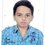# KVS RMO 2009 Q2

I spent around an hour to solve this one:

Let $$a, b, c, d, e$$ be positive real numbers. Prove that

$\large\ \frac { a }{ b+c } +\frac { b }{ c+d } +\frac { c }{ d+e } +\frac { d }{ e+a } +\frac { e }{ a+b } \ge \frac { 5 }{ 2 }$

I did this by applying Titu's lemma:

$\large\ \frac { a }{ b+c } +\frac { b }{ c+d } +\frac { c }{ d+e } +\frac { d }{ e+a } +\frac { e }{ a+b } =\frac { { a }^{ 2 } }{ ab+ac } +\frac { { b }^{ 2 } }{ bc+bd } +\frac { { c }^{ 2 } }{ cd+ce } +\frac { { d }^{ 2 } }{ de+ad } +\frac { { e }^{ 2 } }{ ae+be } \ge \frac { { (a+b+c+d+e })^{ 2 } }{ \sum { ab } }$

Because $\large\ { (a+b+c+d+e })^{ 2 }=\sum { { a }^{ 2 } } +\quad \sum { ab }$,

we have to prove that $\large\ 2\sum { { a }^{ 2 } } +\quad 4\sum { ab\quad \ge } \quad 5\sum { ab, }$

which is equivalent to $\large\ 2\sum { { a }^{ 2 } } \ge \quad \sum { ab }$.

The last inequality follows from $\large\ \sum { { (a-b) }^{ 2 } } \ge \quad 0.$

How is it?

Please do reply and post solutions if any.

.Note by Priyanshu Mishra
5 years, 8 months ago

This discussion board is a place to discuss our Daily Challenges and the math and science related to those challenges. Explanations are more than just a solution — they should explain the steps and thinking strategies that you used to obtain the solution. Comments should further the discussion of math and science.

When posting on Brilliant:

• Use the emojis to react to an explanation, whether you're congratulating a job well done , or just really confused .
• Ask specific questions about the challenge or the steps in somebody's explanation. Well-posed questions can add a lot to the discussion, but posting "I don't understand!" doesn't help anyone.
• Try to contribute something new to the discussion, whether it is an extension, generalization or other idea related to the challenge.
• Stay on topic — we're all here to learn more about math and science, not to hear about your favorite get-rich-quick scheme or current world events.

MarkdownAppears as
*italics* or _italics_ italics
**bold** or __bold__ bold
- bulleted- list
• bulleted
• list
1. numbered2. list
1. numbered
2. list
Note: you must add a full line of space before and after lists for them to show up correctly
paragraph 1paragraph 2

paragraph 1

paragraph 2

[example link](https://brilliant.org)example link
> This is a quote
This is a quote
    # I indented these lines
# 4 spaces, and now they show
# up as a code block.

print "hello world"
# I indented these lines
# 4 spaces, and now they show
# up as a code block.

print "hello world"
MathAppears as
Remember to wrap math in $$ ... $$ or $ ... $ to ensure proper formatting.
2 \times 3 $2 \times 3$
2^{34} $2^{34}$
a_{i-1} $a_{i-1}$
\frac{2}{3} $\frac{2}{3}$
\sqrt{2} $\sqrt{2}$
\sum_{i=1}^3 $\sum_{i=1}^3$
\sin \theta $\sin \theta$
\boxed{123} $\boxed{123}$

## Comments

There are no comments in this discussion.

×

Problem Loading...

Note Loading...

Set Loading...